# Asymptotic Notation

Suppose we have two methods we wish to compare. We have determined that the running time for method A is 10n2 5 milliseconds to process n elements, while that for method B is 100n + 200 milliseconds. (We will discus how to arrive at these expressions in Section 7.3.) Which method should we prefer?

The answer depends on the value of n. As seen in Figure 7-3, for n = 6 method A is faster, but for n = 16 method B is much faster.

Figure 7-3. Method A is faster than method B for small values of n, but slower for large values.

(This item is displayed on page 187 in the print version)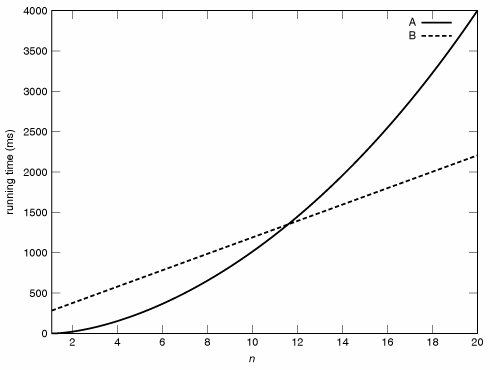We would prefer to say that one method is faster in general, rather than for some particular value of n. The time differences for small values of n are relatively insignificant. What really concerns us is the asymptotic behavior of the running-time functions: what happens as n becomes very large?

Figure 7-3 suggests that the running time for method A is larger than that for method B. If n is at least 12, B is faster.

Our intuition is correct in this example, but graphs can be deceiving. If we had plotted the graph only up to n = 6 (Figure 7-4), we might have concluded that method A is faster. To be confident in our statement about which method is faster for large values of n, we need a proof.

Figure 7-4. If the graph is plotted at a different scale, method A looks better.

(This item is displayed on page 188 in the print version)Theorem: 10n2 5 > 100n + 200 for any value of n [Image_Link]/images/U2265.jpg border=0> 12.

Proof: For any n [Image_Link]/images/U2265.jpg border=0> 12: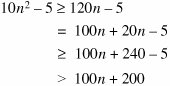The first and third inequalities follow because of the assumption that n [Image_Link]/images/U2265.jpg border=0> 12. This completes the proof.

Actual running times will depend on a number of factors that don't really concern us, including the speed of the hardware, the quality of the compiler, and the skill of the programmer. We would prefer to make statements about the speed of an algorithm in general, rather than a particular implementation of it. This way, we don't have to redo our analysis if we change programming languages or buy a faster computer.

To keep our running-time expressions general, we allow them to contain unspecified constants. For example, we might find that the running time for algorithm A is an2 + b, while that for algorithm B is cn + d, with a, b, c, and d being unspecified constants that depend on factors such as the speed of the hardware.

The thought of doing proofs with all these unspecified constants lying around may be unnerving. Fortunately, there is a huge shortcut. Functions can be classified into orders. (Whenever we talk about orders, we assume that all of the functions involved map nonnegative integers onto nonnegative real numbers and are monotonically nondecreasingthat is, f(n + 1)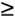n). An algorithm for which the running-time function did not fit into this category would be fairly strange.)

Some common orders are shown in Figure 7-5. We will give a formal definition of an order later in this section.

Figure 7-5. Some common orders of functions. Q is the upper-case Greek letter theta. There are other orders (see page 191), but these are the most frequently encountered.

(This item is displayed on page 189 in the print version)

Order

Nickname

Q(2n)

Q(n3)

cubic

Q(n2)

Q(n log n)

Q(n)

linear

Q(log n)

logarithmic

Q(1)

constant

For any function f, Q(f) is pronounced "the order of f" or simply "order f." Each order is an infinitely large set of functions. The name of the order indicates one of the functions in that order. For example, n2 is one of the functions in Q(n2).

Among the orders in Figure 7-5, Q(2n) is the largest and Q(1) the smallest. This is what makes orders so useful: for sufficiently large n, a function is asymptotically larger than any function in a lower order. For example, for sufficiently large n, a function in Q(n log n) is asymptotically larger than any function in Q(n) and asymptotically smaller than any function in Q(n2).

Two rules go a long way toward identifying the order of a function:

• Multiplying or dividing a function by a positive constant doesn't change its order. For example, 3n2 and 0.2n2 are both in Q(n2).
• A function's order is not changed by adding or subtracting a function in a lower order. For example, 2n n + log n is in Q(2n).

Let's use these rules to find the order of f(n) = 5n3 + 3n2 4n + 11.

By the first rule, 5n3 is in Q(n3). Similarly, 3n2 is in Q(n2), 4n is in Q(n), and 11 is in Q(1). These are all lower orders, so we can ignore them by the second rule. Therefore, f(n) is in Q(n3). This was hardly any work at all, and we now know a great deal about how f(n) compares with other functions.

Let's look at some more examples. Given the running-time expressions in Figure 7-6, which algorithm should we use?

Figure 7-6. Running-time expressions for two fictitious algorithms with silly names.

Algorithm

Running Time

Column frobbing

an2 b log n

Row zorching

cn + d

The running time for the column frobbing algorithm is in Q(n2). The running time for the row zorching algorithm is in Q(n). Q(n) is a lower order, so we should choose row zorching.

Figure 7-7 presents two more running times to compare. Dynamic inversion takes time in Q(n3), but what about synchronized absquatulation?

Figure 7-7. More running-time expressions. The algorithm names don't mean anything.

Algorithm

Running Time

Dynamic inversion

an3 + bn2 + c

Synchronized absquatulation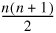Multiplying it out, we find that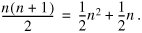Now we are back in familiar territory: Synchronized absquatulation takes time in Q(n2), so it is faster than dynamic inversion (for sufficiently large n).

A final challenge is given in Figure 7-8.

Figure 7-8. Even yet still more running-time expressions. n!, pronounced "n factorial," is the product of the integers 1 through n.

Algorithm

Running Time

Cubic flensing

an3

Factorial decortication

n!

Can we wrangle n! into some form we recognize?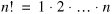Things don't look good. Notice, however, that this is at least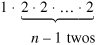or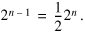Factorial decortication takes time which is at least in Q(2n). Since cubic flensing is in the lower order Q(n3), we should choose cubic flensing.

Rather than proving that Q(n!) = Q(2n), which is not true, we proved something like Q(n!)Q(f) is the set of functions which grow like f, then W(f) is the set of functions which grow like f or much more quickly. In set theory terms, it is the union of Q(f) and all higher orders. We proved that n! is in W(2n). Various related notations are summarized in Figure 7-9.

Figure 7-9. Order relations between two functions f and g. The symbolis read "is a member of (the set)." g) might actually be larger than g.

Analogy

Notation

Set

Q(f)fQ(g) and all higher orders

Q(f) = Q(g)

fQ(g)

Q(f)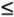fO(

Q(g) and all lower orders

Remember that functions in the same order can differ by a constant factor. Consequently, a function in O(g) might actually be larger than g. For example, 3n2O(n2 is larger than n2 (by a factor of 3).

We can define these notations more formally. First, fO(c > 0, there is some n00 such that n) < cg(n) for any nWe've seen n0 before; that's just a threshold so that only large values of n are considered. (In Figure 7-3, n0 was 12.) The constant c is there so that multiplying either function by a constant won't have any effect. Put another way, the definition says that fO(Q(g) is larger than f for sufficiently large n.

Similarly, f0 such that n) > cg(n) for any nCombining these, we get the formal definition of an order:

fO(fO(asymptotic upper bound on f. Showing that fQ(kn) are called exponential orders. For k > 0, the orders Q(nk) are called polynomial orders. These fit into the order hierarchy right where we would expect. For example, n5O(3in7.2

What is the order of the expression 3n2 + 5n + n log n?

7.3

What is the order of the expression 5n log 5n?

7.4

What is the order of the expression (n + 3)(n 2)?

7.5

What is the order of the expression7.6

What is the order of the volume of an n x n x n cube? What about the surface area? Answer the same questions for a cylinder of radius n and height n.

7.7

Use the identity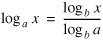to prove that Q(log2 n), Q(log10 n), and Q(loge n) are the same order.

7.8

Prove that Q(max(f, g)) = Q(f + g). (Hint: Since max(f, g) = max(g, f) and f + g = g + f, you may assume without loss of generality that f(n) is larger than g(n) for sufficiently large values of n.)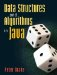Data Structures and Algorithms in Java
ISBN: 0131469142
EAN: 2147483647
Year: 2004
Pages: 216
Authors: Peter Drake